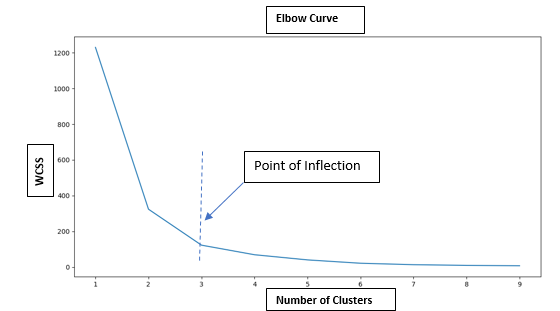....

January 16, 2021

# What is the significance of ‘K’ in K-Means and how is it calculated?

K in K-Means clustering signifies the number of clusters. In K-Means algorithm we need to specify the value of K. As is the inherent nature of the algorithm, with increase in number of clusters the WCSS decreases. But the rate of decrease is steep for first few points and then plateaus. The point of inflection is the ideal number of cluster for the given dataset i.e. the ideal value of K.by : Monis Khan

Quick Summary:

K in K-Means clustering signifies the number of clusters. In K-Means algorithm we need to specify the value of K. As is the inherent nature of the algorithm, with increase in number of clusters the WCSS decreases. But the rate of decrease is steep for first few points and then plateaus. The point of inflection […]